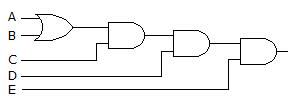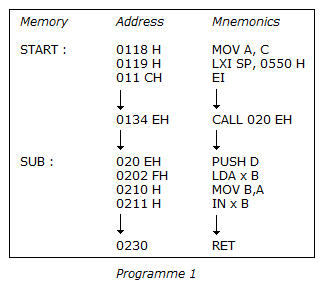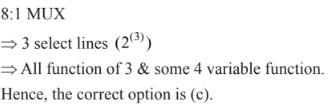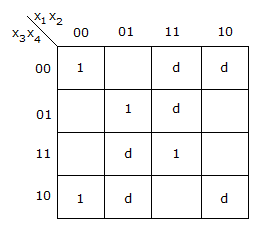# Test: Digital Electronics - 6

## 25 Questions MCQ Test Digital Electronics | Test: Digital Electronics - 6

Description
This mock test of Test: Digital Electronics - 6 for Electrical Engineering (EE) helps you for every Electrical Engineering (EE) entrance exam. This contains 25 Multiple Choice Questions for Electrical Engineering (EE) Test: Digital Electronics - 6 (mcq) to study with solutions a complete question bank. The solved questions answers in this Test: Digital Electronics - 6 quiz give you a good mix of easy questions and tough questions. Electrical Engineering (EE) students definitely take this Test: Digital Electronics - 6 exercise for a better result in the exam. You can find other Test: Digital Electronics - 6 extra questions, long questions & short questions for Electrical Engineering (EE) on EduRev as well by searching above.
QUESTION: 1

Solution:
QUESTION: 2

Solution:
QUESTION: 3

### 2's complement representation of a 16 bit number (one sign bit and 15 magnitude bit) is FFFF. Its magnitude in decimal representation is

Solution:
QUESTION: 4

Which one of the following is the correct sequence of the numbers represented in the series given below?

Solution:
QUESTION: 5

A 4 line to 16 line decoder has

Solution:
QUESTION: 6

Derive the Boolean expression for the logic circuit shown below:Solution:
QUESTION: 7

In floating point representation the number of bits in exponent is

Solution:
QUESTION: 8

Consider the following statements:

1. In the 2's complement representation, negative numbers are stored in sign magnitude form.
2. Taking 2's complement is equivalent to sign change.
3. In a 4 bit complement representation of a binary number A.
4. In the 2's complement representation the most significant bit (MSB) is zero for a positive number.
Of these, the only true statements are
Solution:
QUESTION: 9

In 8085 microprocessor, an active low signal INTA is not needed from up service which of the following interrupt request?Solution:
QUESTION: 10

Without any addition circuitry, an 8:1 MUX can be used to obtain.

Solution:QUESTION: 11

The internal structure of PL A is similar to

Solution:
QUESTION: 12

Start and stop bit do not contain any information but are used in serial communication for

Solution:
QUESTION: 13

Which of the following parameters is not specified for digital ICs?

Solution:
QUESTION: 14

Solution:
QUESTION: 15

Program counter in a digital computer

Solution:
QUESTION: 16

Which are the pending interrupts?

Solution:
QUESTION: 17

The logical expression Y = A+AB is equivalent to

Solution:
QUESTION: 18

A quantization error is an essential aspect of ADC

Solution:
QUESTION: 19

Fundamental mode is another name for

Solution:
QUESTION: 20

Schmitt trigger is used for wave shaping.

Solution:
QUESTION: 21

Assertion (A): Decoder is not a combinational circuit.

Reason (R): Every memory element of semi conductor form has a decoder.

Solution:
QUESTION: 22

A(A + B) =

Solution:
QUESTION: 23

In 8085 microprocessor, in order to enable RST 5.5, RST 6.5 and RST 7.5 interrupts, which of the following instruction are needed?

Solution:
QUESTION: 24

A retriggerable one shot is one which

Solution:
QUESTION: 25

For the K map in the given figure, the simplified expression isSolution: